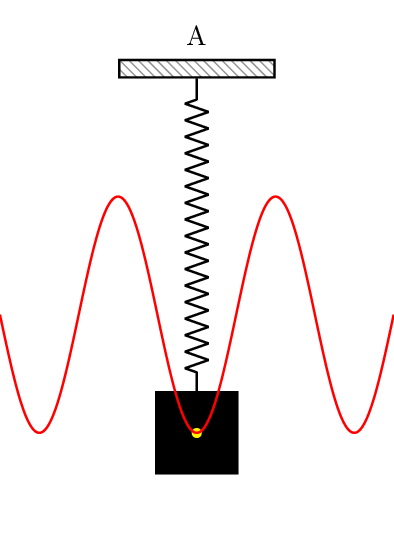# Periodic Motion

Periodic motion repeats itself at regular intervals of time. Examples of periodic are the swinging of a pendulum, the motion of a mass attached to a spring, and the oscillation of a tuning fork.The motion is described by specifying the position $x$ and velocity $\vec{v}$ at time $t$. The position and velocity of the particle attains the same value after a regular time interval. This time interval is called time period ($T$). The motion for time $T$ is called a cycle.

The frequency $\nu$ is the number of cycles in unit time (say one second). It is related to the time period by \begin{align} \nu=\frac{1}{T}. \end{align}

The amplitude $A$ of a periodic motion is the maximum displacement of the object from its equilibrium (or mean) position.

## Problems from IIT JEE

Problem (JEE Mains 2021): Time period of a simple pendulum is $T$. The time taken to complete 5/8 oscillations starting from mean position is $\frac{\alpha}{\beta}T$. The value of $\alpha$ is_________